A Worked Example using Graphics Primitives: The Wulff Construction

This next example shows a clever way to perform a famous thermodynamic graphical construction called  the  Wulff construction.  For surface tensions that are a function of their orientation, the Wulff construction gives the enclosing surface of lowest total energy.  The following code creates the graphics objects corresponding to the Wulff construction; it will be combined with an orientation-dependent surface tension to produce a prediction of the energy-minimizing shape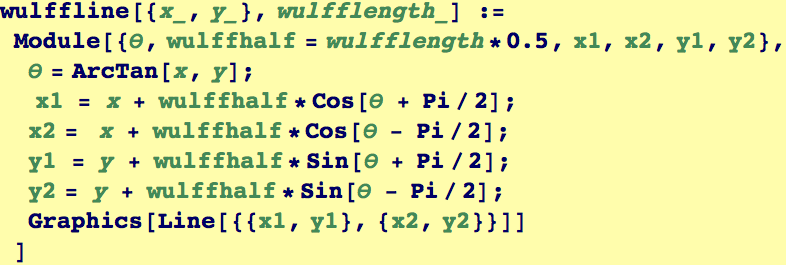These last two plots have to do with the orientation dependence of the surface energy of a 2-D crystal (GammaPlot)and the equilibrium shape that a single crystal having this surface energy will adopt to minimize its total surface energy.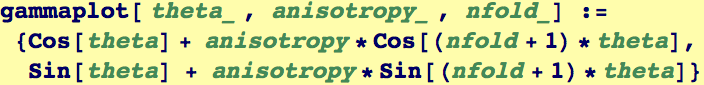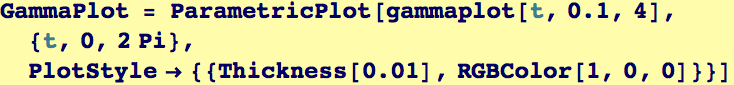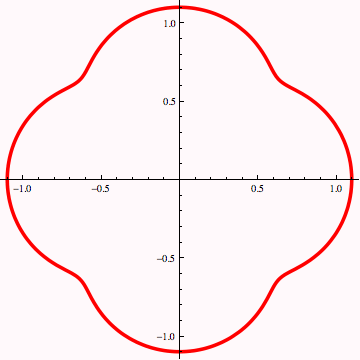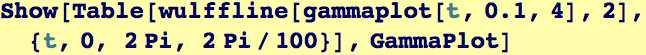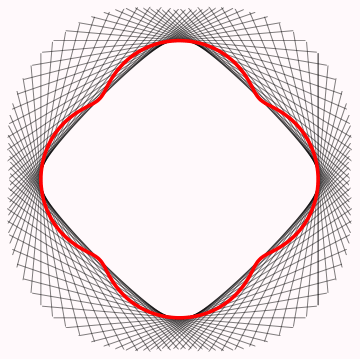The inner envelope of the tangent lines to the GammaPlot in the figure above illustrates the energy-minimizing shape of the crystal. For this particular GammaPlot, the shape is approximately a square but the corners are rounded.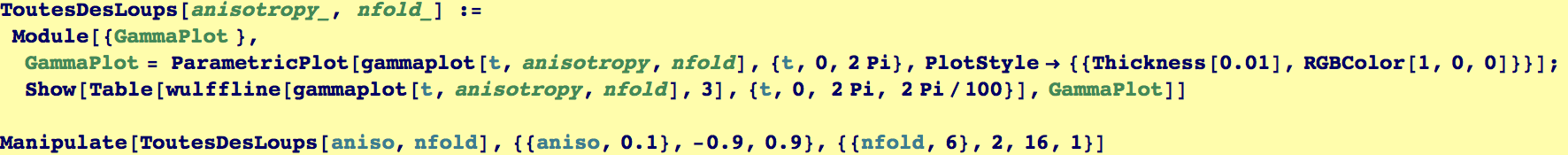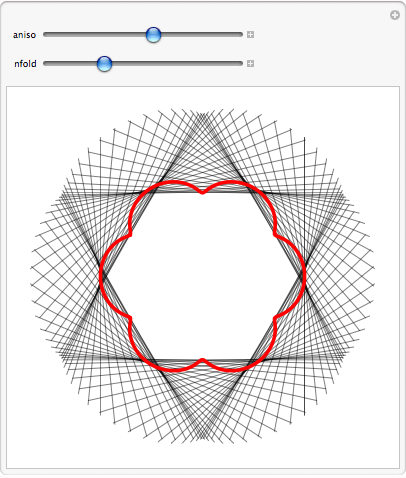Created by Wolfram Mathematica 6.0  (28 August 2007)